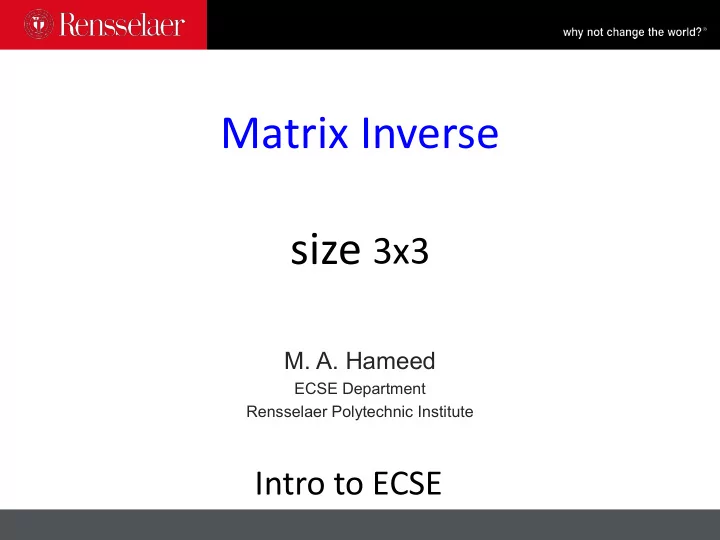# Is the matrix invertible? Matrix A nxn is invertible if there exists - PowerPoint PPT Presentation

## Matrix Inverse size 3x3 M. A. Hameed ECSE Department Rensselaer Polytechnic Institute Intro to ECSE Is the matrix invertible? Matrix A nxn is invertible if there exists B nxn such that AB = BA = I n Invertible = nonsingular =

1. Matrix Inverse size 3x3 M. A. Hameed ECSE Department Rensselaer Polytechnic Institute Intro to ECSE

2. Is the matrix invertible? • Matrix A nxn is invertible if there exists B nxn such that AB = BA = I n • Invertible = nonsingular = nondegenerate • Non-Invertible = singular = degenerate • Matrix A is invertible if and only if det 𝑩 ≠ 0 M. Hameed 7 October 2019

3. Invert Z 3x3 to determine Z -1 𝑏 𝑐 𝑑 𝑒 𝑓 𝑔 𝒂 = 𝑕 ℎ 𝑗 3×3 Transpose ④ 𝑈 ' 𝐵 𝐶 𝐷 1 1 𝒂 −1 = det 𝒂 𝑏𝑒𝑘 𝒂 = 𝐸 𝐹 𝐺 det 𝒂 𝐻 𝐼 𝐽 𝑏𝑒𝑘 𝒂 = Transpose of matrix of cofactors M. Hameed 7 October 2019

4. 𝑏 𝑐 𝑑 𝑈 𝐵 𝐶 𝐷 𝑒 𝑓 𝑔 𝒂 = 𝑏𝑒𝑘(𝒂) = 𝐸 𝐹 𝐺 * 𝑕 ℎ 𝑗 𝐻 𝐼 𝐽 𝐵 = 𝑓𝑗 − 𝑔ℎ 𝐶 = − 𝑒𝑗 − 𝑔𝑕 𝐷 = 𝑒ℎ − 𝑓𝑕 𝐸 = − 𝑐𝑗 − 𝑑ℎ 𝐹 = 𝑏𝑗 − 𝑑𝑕 𝐺 = − 𝑏ℎ − 𝑐𝑕 𝐻 = 𝑐𝑔 − 𝑑𝑓 𝐼 = − 𝑏𝑔 − 𝑑𝑒 𝐽 = 𝑏𝑓 − 𝑐𝑒 + t - det 𝒂 = 𝑏𝐵 + 𝑐𝐶 + 𝑑𝐷 f - - t t - M. Hameed 7 October 2019

5. Example ' E = ? −1 1 0 Firstling : 𝒂 = 2 1 −1 1 2 −2 = E 3 X 3 =L ; ; I ;] ÷ adic ⇒ . . ÷ ;¥÷ ¥ , den ⇒ :B M. Hameed 7 October 2019 7 October 2019

6. Che4 - ' = I z Z . M. Hameed 7 October 2019

More recommend